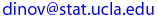Ivo Dinov
UCLA Statistics, Neurology, LONI
, Math/PIC
 Courses SOCR Ivo Dinov's Home SiteMap Software Contact

STAT 35
Winter  2005

Interactive and Computational Probability

Department of Statistics

## Instructor: Ivo Dinov

Homework 5
Due Date: Monday, Mar. 14, 2005

Please, submit your homework right after lecture on the due date. See the HW submission rules. On the front page include the following header.

• (HW_5_1) Consider writing onto a computer disk and then sending it through a certifier that counts X, the number of missing pulses. Suppose this number X has a Poisson distribution with parameter λ = 0.2. Run the Poisson Experiment under the SOCR Experiments and calcualte emperically (by running 100 or more experiments) and theoretically (by using the Poisson probability formula) each of the following probabilities.

• (a) The probability that a disk has exactly one missing pulse?
(b) The probability that a disk has at least two missing pulses?
(c) If two disks are independently selected, what is the probability that neither contains a missing pulse?

• (HW_5_2) The Pareto family of distributions (see SOCR Distributions) has been used to approximate the distribution of income, city population size, size of firms, among others. The Pareto distribution has two parameters, k and ϑ, both strictly positive, and its pdf is
 | kϑk / xk+1 x≥ϑ f(x; k, ϑ) = | | 0 x<ϑ

•   (a) Describe the effects of the two parameters on the shape of the graph of f(x; k, ϑ).
(b) Verify that the total area under the for all equals 1.
(c) If the R.V. X has Pareto Distribution f(x; k, ϑ) and b > ϑ obtain an expression for the CDF F(b) = P(X ≤ b).
(d) For ϑ < a < b, obtain an expression for the probability P(a ≤ X ≤ b) = F(b) − F(a).
(e) If k > 1, compute E(X).
(f) What are the expected value, E(X), and the standard deviation, SD(X), if k = 1 and ϑ=4? Validate your closed-form mathematical expression by using the Pareto Distribution Modeler.

• (HW_5_3)  Suppose the diameter at breast height of trees (in.) of a certain type is Normally distributed with µ = 8.8 and σ = 2.8. Use the Normal Distribution Modeler part of SOCR Distributions to compute the following probabilities.

•   (a) What is the probability that the diameter of a randomly selected tree will be at least 10 in.? Will exceed 10 in.?
(b) What is the probability that the diameter of a randomly selected tree will exceed 20 in.?
(c) What is the probability that the diameter of a randomly selected tree will be between 5 and 10 in.?
(d) What value c is such that the interval (8.8−c, 8.8+c) includes 98% of all diameter values?

• (HW_5_4) The Rockwell hardness of a metal is determined by impressing a hardened point into the surface of the metal and then measuring the depth of penetration of the point. Suppose the Rockwell hardness of a particular alloy is measured on a continuous scale and is Normally distributed with mean 70 and standard deviation 3. Use the Normal Distribution Modeler part of SOCR Distributions to compute the following:

•   (a) If a specimen is acceptable only if its hardness is between 67 and 75, what is the chance that a randomly chosen specimen has an acceptable hardness?
(b) If the acceptable range of hardness is (70−c, 70+c), for what value of c would 95% of all specimens have acceptable hardness?
(c) If the acceptable range is as in part (a) and the hardness of each of ten randomly selected specimens is independently determined, what is the expected number of acceptable specimens among the ten?

 Ivo Dinov's Home http://www.stat.ucla.edu/~dinov Visitor number, since Jan. 01, 2002 © I.D.Dinov 1997-2005
Last modified on by